Courses

# Engineering Mathematics - 2

## 10 Questions MCQ Test GATE Computer Science Engineering(CSE) 2022 Mock Test Series | Engineering Mathematics - 2

Description
This mock test of Engineering Mathematics - 2 for Computer Science Engineering (CSE) helps you for every Computer Science Engineering (CSE) entrance exam. This contains 10 Multiple Choice Questions for Computer Science Engineering (CSE) Engineering Mathematics - 2 (mcq) to study with solutions a complete question bank. The solved questions answers in this Engineering Mathematics - 2 quiz give you a good mix of easy questions and tough questions. Computer Science Engineering (CSE) students definitely take this Engineering Mathematics - 2 exercise for a better result in the exam. You can find other Engineering Mathematics - 2 extra questions, long questions & short questions for Computer Science Engineering (CSE) on EduRev as well by searching above.
QUESTION: 1

### Find the value of x for the singular matrix?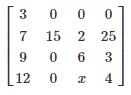Solution:

Determinant of a singular matrix is 0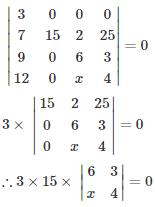∴ 3×15×(24−3x)=0
∴ 3x=24
∴ x=8

*Answer can only contain numeric values
QUESTION: 2

### If the determinant of the matrix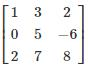is 26, then the determinant of the matrix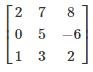is

Solution:

If two rows are interchanged in a determinant then the value of the determinant does not change but sign will change. In the given question, first and third rows are interchanged.

Determinant wil be -26

QUESTION: 3

### The value of the determinant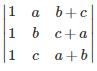Solution: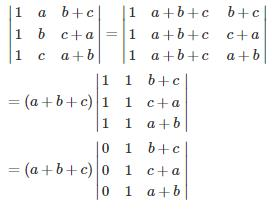QUESTION: 4

If a matrix A is given by f(x) = a0 + a1x + a2x2+…+ an−1xn−1 + anxn, then the determinant of A is

Solution:

matrix ‘A’ ⇒ f(x) = a+ a1x + a2x+……….+an−1xn−1 + anxnf(x)

if 2 × 2 matrix then characteristic equation  ⇒ aλ+ bλ + c = 0
⇒ |A| = λ12. = c/a
where ao = c;an = a2 = a
if 3 × 3 matrix → then characteristic equation ⇒ aλ+ bλ+ cλ + d = 0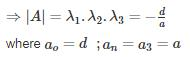∴ For n × n matrix, |A| = λ123….λn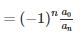QUESTION: 5

What is the determinant of the below-given matrix?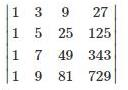Solution:

Determinant of the given matrix is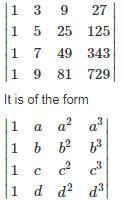Δ = (a − b) (a − c) (a − d) (b − c) (b − d)(c − d)

a = 3, b = 5, c = 7, d = 9

∴ Δ = 768

QUESTION: 6

If  A =  then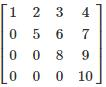the value of adj (adj (A))?

Solution: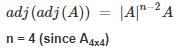A is upper triangular matrix

∴ product of diagonal elements = |A|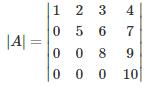= 1 × 5 × 8 × 10 = 400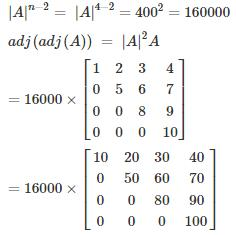Note:

|A||A| is determinant of matrix A

*Answer can only contain numeric values
QUESTION: 7

What is the rank of A−B where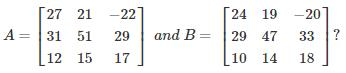Solution: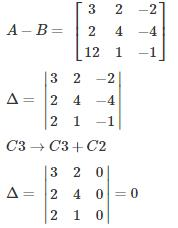∴ rank is < 3

Also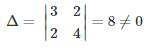Hence rank of is A−B is 2

QUESTION: 8

What is the value of A− 9A− 47A?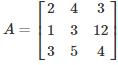Solution:

Characteristic equation of the given matrix is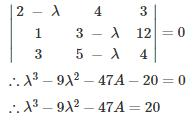Every matrix satisfies its own characteristic equation

∴A3 − 9A2 − 47A = 20I

QUESTION: 9

The factorized form of the following determinant is: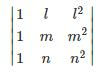Solution:Applying R2 → R2 – R1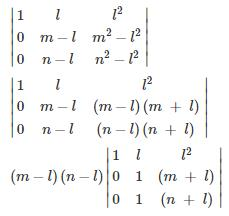Now, expanding from a11.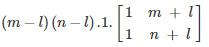= (m - l)(n - l)(n + l - m - l)

= (m - l)(n - l)(n - m)

QUESTION: 10

A 3 × 5 matrix has all its entries equal to 1. The rank of the matrix is

Solution:

Rank ≤ 3 since all entries are 1,

∴| 3 × 3 submatrices | = | 2 × 2 submatrices | = 0

only 1 × 1 or single entries are ≠ 0

∴ rank = 1